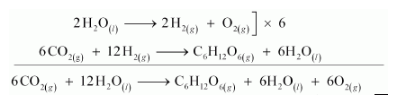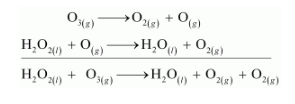# Consider the reactions:

Question:

Consider the reactions:

(a) $6 \mathrm{CO}_{2}(\mathrm{~g})+6 \mathrm{H}_{2} \mathrm{O}(\mathrm{l}) \rightarrow \mathrm{C}_{6} \mathrm{H}_{12} \mathrm{O}_{6}(\mathrm{aq})+6 \mathrm{O}_{2}(\mathrm{~g})$

(b) $\mathrm{O}_{3}(\mathrm{~g})+\mathrm{H}_{2} \mathrm{O}_{2}(\mathrm{l}) \rightarrow \mathrm{H}_{2} \mathrm{O}(\mathrm{l})+2 \mathrm{O}_{2}(\mathrm{~g})$

Why it is more appropriate to write these reactions as:

(a) $6 \mathrm{CO}_{2}(\mathrm{~g})+12 \mathrm{H}_{2} \mathrm{O}(\mathrm{l}) \rightarrow \mathrm{C}_{6} \mathrm{H}_{12} \mathrm{O}_{6}($ aq $)+6 \mathrm{H}_{2} \mathrm{O}(\mathrm{I})+6 \mathrm{O}_{2}(\mathrm{~g})$

(b) $\mathrm{O}_{3}(\mathrm{~g})+\mathrm{H}_{2} \mathrm{O}_{2}(\mathrm{I}) \rightarrow \mathrm{H}_{2} \mathrm{O}(\mathrm{I})+\mathrm{O}_{2}(\mathrm{~g})+\mathrm{O}_{2}(\mathrm{~g})$

Also suggest a technique to investigate the path of the above (a) and (b) redox reactions.

Solution:

(a)The process of photosynthesis involves two steps.

Step 1:

$\mathrm{H}_{2} \mathrm{O}$ decomposes to give $\mathrm{H}_{2}$ and $\mathrm{O}_{2}$.

$2 \mathrm{H}_{2} \mathrm{O}_{(t)} \longrightarrow 2 \mathrm{H}_{2(\mathrm{z})}+\mathrm{O}_{2(\mathrm{~g})}$

Step 2:

The $\mathrm{H}_{2}$ produced in step 1 reduces $\mathrm{CO}_{2}$, thereby producing glucose $\left(\mathrm{C}_{6} \mathrm{H}_{12} \mathrm{O}_{6}\right)$ and $\mathrm{H}_{2} \mathrm{O}$.

$6 \mathrm{CO}_{2(s)}+12 \mathrm{H}_{2(s)} \longrightarrow \mathrm{C}_{6} \mathrm{H}_{12} \mathrm{O}_{6(s)}+6 \mathrm{H}_{2} \mathrm{O}_{(\prime)}$

Now, the net reaction of the process is given as:It is more appropriate to write the reaction as given above because water molecules are also produced in the process of photosynthesis.

The path of this reaction can be investigated by using radioactive $\mathrm{H}_{2} \mathrm{O}^{18}$ in place of $\mathrm{H}_{2} \mathrm{O}$.

(b) $\mathrm{O}_{2}$ is produced from each of the two reactants $\mathrm{O}_{3}$ and $\mathrm{H}_{2} \mathrm{O}_{2}$. For this reason, $\mathrm{O}_{2}$ is written twice.

The given reaction involves two steps. First, $\mathrm{O}_{3}$ decomposes to form $\mathrm{O}_{2}$ and $\mathrm{O}$. In the second step, $\mathrm{H}_{2} \mathrm{O}_{2}$ reacts with the $\mathrm{O}$ produced in the first step, thereby producing $\mathrm{H}_{2} \mathrm{O}$ and $\mathrm{O}_{2}$.The path of this reaction can be investigated by using $\mathrm{H}_{2} \mathrm{O}_{2}{ }^{18}$ or $\mathrm{O}_{3}^{18}$.